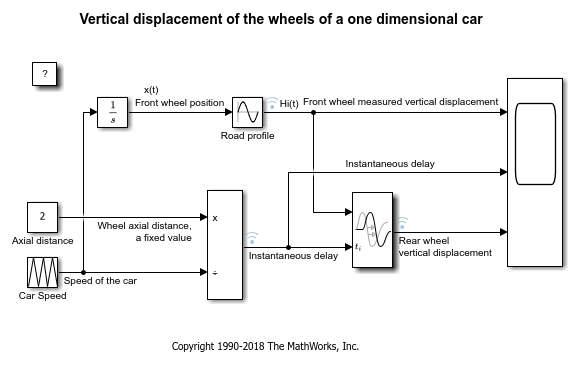# Saving and Restoring Simulation Operating Point

This example shows the workflow and the benefits of saving and restoring a simulation operating point using a ModelOperatingPoint object.

The ModelOperatingPoint object contains the complete snapshot of all variables that are related to the simulation of a model. After saving the ModelOperatingPoint object at the end of a simulation, Simulink® can reload the operating point and continue the simulation from the time at which the ModelOperatingPoint object was saved. This action produces the same simulation results as if the simulation were never interrupted.

This example illustrates:

• How saving the Final states (logged states) is not always sufficient for complete and accurate restoration of a simulation state.

• How you can save and restore the complete operating point of a simulation, yielding faster simulation workflows

### Difficulties with Models with Variable Transport Delay

The states that are logged during a simulation are a subset of all the information needed to fully describe the state of a simulation. Certain blocks rely on internal information that is not logged as part of state logging and final state export. One such block is the Transport Delay block. Models with Transport Delay blocks are usually difficult to fully restore to a particular state because the state of the transport delay is not saved in the structure format or the array format as part of "Final states" data logging.

To illustrate this issue, compare the simulation results for two cases:

1. Simulate a model, which contains a Transport Delay block, from 0 to 5 seconds and save the "Final states" values in the workspace. Then load this first set of final states and simulate from 5 to 10 seconds.

2. Simulate the same model from 0 to 10 seconds and force the model to produce an output at 5 seconds. We call the result of this simulation the baseline result because it is a nonstop simulation.

The results of the first simulation match the first half of the baseline result. If the simulation state of the first simulation had been restored completely, the second simulation results would match the second half of the baseline.

```mdl = 'slexVariableTransportDelay'; load_system(mdl); ```

Simulate until time of 5 and save the final state in structure format:

```out = sim(mdl, 'StopTime', '5', 'SaveFinalState', 'on',... 'FinalStateName','xFinal', 'SaveFormat','Structure'); y1 = out.ScopeData; ```

Load the final state from the last simulation and run to 10:

```assignin('base', 'xFinal', out.get('xFinal')); out1 = sim(mdl, 'StartTime', '5', 'StopTime', '10', ... 'SaveFinalState', 'off', ... 'LoadInitialState', 'on', 'InitialState', 'xFinal'); y2 = out1.ScopeData; ```

Run a nonstop simulation which will serve as the baseline result:

```out2 = sim(mdl, 'OutputOption', 'AdditionalOutputTimes' ,... 'OutputTimes','[0 5 10]', 'LoadInitialState', 'off'); y = out2.ScopeData; ```

Plot the results. Note that the second half of the baseline result does not match the simulation from 5 to 10 seconds, whose initial state was restored from the final state saved at 5 seconds:

```figure; for idx=1:3 subplot(3, 1, idx); plot(y.time,y.signals(idx).values); hold on; plot([y1.time; y2.time],... [y1.signals(idx).values;y2.signals(idx).values],'r--'); hold off; grid on; end ```### Saving and Restoring the Complete Operating Point of a Simulation

You can save the complete final operating point in a Simulink.op.ModelOperatingPoint object. The ModelOperatingPoint object contains all the variables which are needed to restore the simulation results. By using the complete ModelOperatingPoint, Simulink is able to restore the simulation state completely and to reproduce the baseline simulation results.

Set the parameter for Simulink to save the complete operating point at the end of the simulation.

```out3 = sim(mdl, 'StopTime', '5', 'SaveFinalState', 'on', ... 'LoadInitialState', 'off', 'SaveOperatingPoint', 'on',... 'FinalStateName', 'xFinal'); y1 = out3.ScopeData; ```

Load the ModelOperatingPoint from the last simulation and run for an additional 5 seconds. The start time value must remain 0.0 (that value was the start time of the original simulation). The software stores the original simulation start time in xFinal. This value must match the start time of the current simulation to enable restoration of the simulation state.

```assignin('base', 'xFinal', out3.get('xFinal')); out4 = sim(mdl, 'StopTime', '10', 'SaveFinalState', 'off', ... 'LoadInitialState', 'on', 'InitialState', 'xFinal'); y2 = out4.ScopeData; ```

Plot the results and compare them with the baseline simulation. Note that this time, the simulation state has been completely restored and the simulation results after the operating point restore match the baseline.

```figure; for idx=1:3 subplot(3, 1, idx); plot(y.time,y.signals(idx).values); hold on plot([y1.time; y2.time],... [y1.signals(idx).values;y2.signals(idx).values],'r--'); hold off; grid on; end ```Close the model and clear the variables which were used in this example

```close_system(mdl, 0); clear mdl idx xFinal y y1 y2 y3 out out1 out2 out3 out4 ```